# 卷起来了！Android OpenGL 仿自如 APP 裸眼 3D 效果

13,367

## 概述## 原理简介 & OpenGL 的优势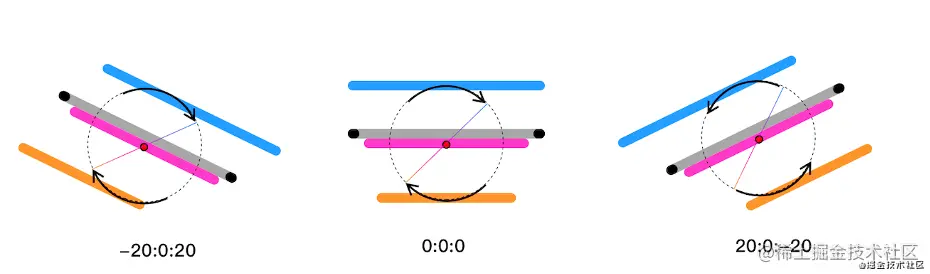## 具体实现

### 1. 绘制静态图片

``````// 顶点着色器代码
// 顶点坐标
attribute vec4 av_Position;
// 纹理坐标
attribute vec2 af_Position;
uniform mat4 u_Matrix;
varying vec2 v_texPo;

void main() {
v_texPo = af_Position;
gl_Position =  u_Matrix * av_Position;
}
``````
``````// 顶点着色器代码
// 顶点坐标
attribute vec4 av_Position;
// 纹理坐标
attribute vec2 af_Position;
uniform mat4 u_Matrix;
varying vec2 v_texPo;

void main() {
v_texPo = af_Position;
gl_Position =  u_Matrix * av_Position;
}
``````

``````public class My3DRenderer implements GLSurfaceView.Renderer {

@Override
public void onSurfaceCreated(GL10 gl, EGLConfig config) {
mContext,
);

// ...

// 2. 依次将3张切图纹理传入GPU
this.texImageInner(R.drawable.bg_3d_back, mBackTextureId);
this.texImageInner(R.drawable.bg_3d_mid, mMidTextureId);
this.texImageInner(R.drawable.bg_3d_fore, mFrontTextureId);
}
}
``````

``````public class My3DRenderer implements GLSurfaceView.Renderer {

// 投影矩阵
private float[] mProjectionMatrix = new float;

@Override
public void onSurfaceChanged(GL10 gl, int width, int height) {
// 设置视口大小，这里设置全屏
GLES20.glViewport(0, 0, width, height);
// 图像和屏幕宽高比基本一致，简化处理，使用一个单位矩阵
Matrix.setIdentityM(mProjectionMatrix, 0);
}
}
``````

``````public class My3DRenderer implements GLSurfaceView.Renderer {

private float[] mBackMatrix = new float;
private float[] mMidMatrix = new float;
private float[] mFrontMatrix = new float;

@Override
public void onDrawFrame(GL10 gl) {
GLES20.glClear(GLES20.GL_COLOR_BUFFER_BIT);
GLES20.glClearColor(0.0f, 0.0f, 0.0f, 1.0f);

GLES20.glUseProgram(mProgram);

// 依次绘制背景、中景、前景
this.drawLayerInner(mBackTextureId, mTextureBuffer, mBackMatrix);
this.drawLayerInner(mMidTextureId, mTextureBuffer, mMidMatrix);
this.drawLayerInner(mFrontTextureId, mTextureBuffer, mFrontMatrix);
}

private void drawLayerInner(int textureId, FloatBuffer textureBuffer, float[] matrix) {
// 1.绑定图像纹理
GLES20.glBindTexture(GLES20.GL_TEXTURE_2D, textureId);
// 2.矩阵变换
GLES20.glUniformMatrix4fv(uMatrixLocation, 1, false, matrix, 0);
// ...
// 3.执行绘制
GLES20.glDrawArrays(GLES20.GL_TRIANGLE_STRIP, 0, 4);
}
}
``````### 2. 让图片动起来

``````// 2.1 注册传感器
mSensorManager = (SensorManager) context.getSystemService(Context.SENSOR_SERVICE);
mAcceleSensor = mSensorManager.getDefaultSensor(Sensor.TYPE_ACCELEROMETER);
mMagneticSensor = mSensorManager.getDefaultSensor(Sensor.TYPE_MAGNETIC_FIELD);
mSensorManager.registerListener(mSensorEventListener, mAcceleSensor, SensorManager.SENSOR_DELAY_GAME);
mSensorManager.registerListener(mSensorEventListener, mMagneticSensor, SensorManager.SENSOR_DELAY_GAME);

// 2.2 不断接受旋转状态
private final SensorEventListener mSensorEventListener = new SensorEventListener() {
@Override
public void onSensorChanged(SensorEvent event) {
// ... 省略具体代码
float[] values = new float;
float[] R = new float;
SensorManager.getRotationMatrix(R, null, mAcceleValues, mMageneticValues);
SensorManager.getOrientation(R, values);
// x轴的偏转角度
float degreeX = (float) Math.toDegrees(values);
// y轴的偏转角度
float degreeY = (float) Math.toDegrees(values);
// z轴的偏转角度
float degreeZ = (float) Math.toDegrees(values);

// 拿到 xy 轴的旋转角度，进行矩阵变换
updateMatrix(degreeX, degreeY);
}
};
``````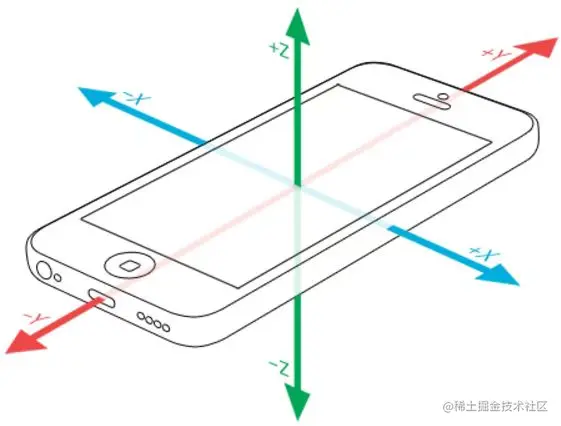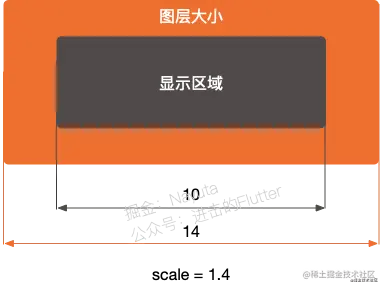``````public class My3DRenderer implements GLSurfaceView.Renderer {

private float[] mBackMatrix = new float;
private float[] mMidMatrix = new float;
private float[] mFrontMatrix = new float;

/**
* 陀螺仪数据回调，更新各个层级的变换矩阵.
*
* @param degreeX x轴旋转角度，图片应该上下移动
* @param degreeY y轴旋转角度，图片应该左右移动
*/
private void updateMatrix(@FloatRange(from = -180.0f, to = 180.0f) float degreeX,
@FloatRange(from = -180.0f, to = 180.0f) float degreeY) {
// ... 其它处理

// 背景变换
// 1.最大位移量
float maxTransXY = MAX_VISIBLE_SIDE_BACKGROUND - 1f;
// 2.本次的位移量
float transX = ((maxTransXY) / MAX_TRANS_DEGREE_Y) * -degreeY;
float transY = ((maxTransXY) / MAX_TRANS_DEGREE_X) * -degreeX;
float[] backMatrix = new float;
Matrix.setIdentityM(backMatrix, 0);
Matrix.translateM(backMatrix, 0, transX, transY, 0f);                    // 2.平移
Matrix.scaleM(backMatrix, 0, SCALE_BACK_GROUND, SCALE_BACK_GROUND, 1f);  // 1.缩放
Matrix.multiplyMM(mBackMatrix, 0, mProjectionMatrix, 0, backMatrix, 0);  // 3.正交投影

// 中景变换
Matrix.setIdentityM(mMidMatrix, 0);

// 前景变换
// 1.最大位移量
maxTransXY = MAX_VISIBLE_SIDE_FOREGROUND - 1f;
// 2.本次的位移量
transX = ((maxTransXY) / MAX_TRANS_DEGREE_Y) * -degreeY;
transY = ((maxTransXY) / MAX_TRANS_DEGREE_X) * -degreeX;
float[] frontMatrix = new float;
Matrix.setIdentityM(frontMatrix, 0);
Matrix.translateM(frontMatrix, 0, -transX, -transY - 0.10f, 0f);            // 2.平移
Matrix.scaleM(frontMatrix, 0, SCALE_FORE_GROUND, SCALE_FORE_GROUND, 1f);    // 1.缩放
Matrix.multiplyMM(mFrontMatrix, 0, mProjectionMatrix, 0, frontMatrix, 0);  // 3.正交投影
}
}
``````

### 3. 几个反直觉的细节

#### 3.1 旋转方向 ≠ 位移方向

``````// 设备旋转方向和图片的位移方向是相反的
float transX = ((maxTransXY) / MAX_TRANS_DEGREE_Y) * -degreeY;
float transY = ((maxTransXY) / MAX_TRANS_DEGREE_X) * -degreeX;
// ...
Matrix.translateM(backMatrix, 0, transX, transY, 0f);
``````

#### 3.2 默认旋转角度 ≠ 0°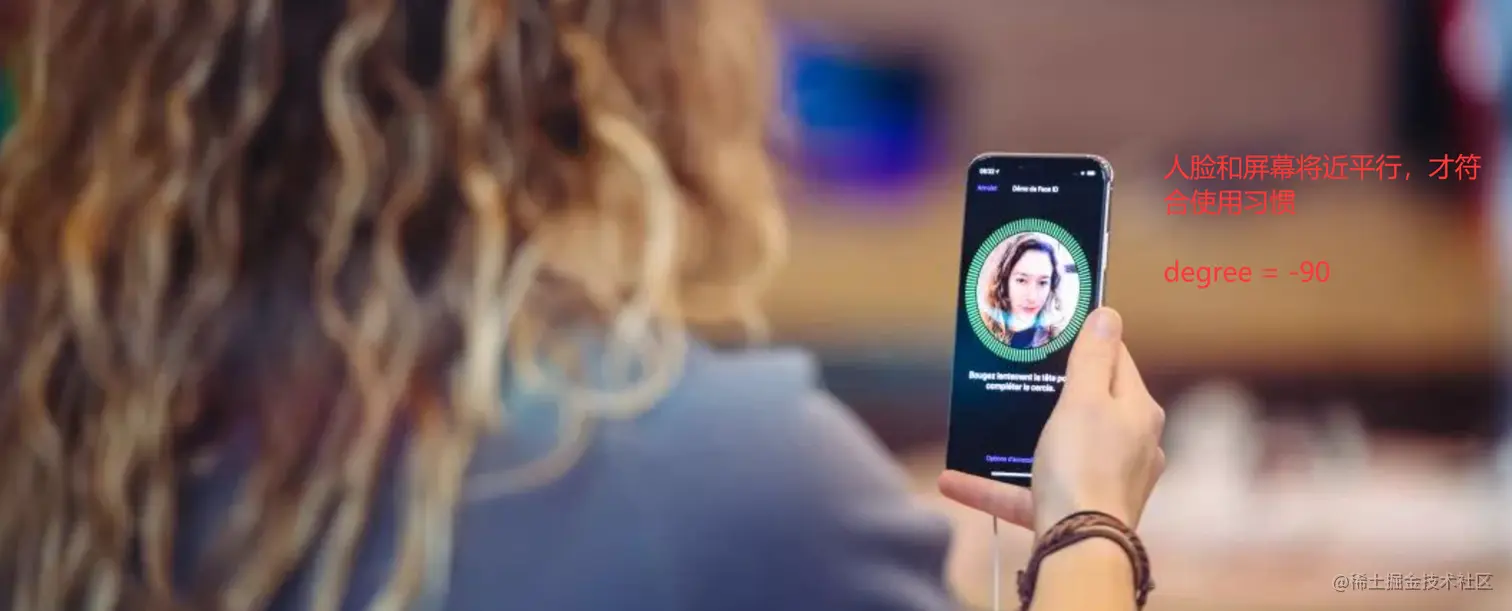``````private static final float USER_X_AXIS_STANDARD = -45f;
private static final float MAX_TRANS_DEGREE_X = 25f;   // X轴最大旋转角度 ∈ (-20°，-70°)

private static final float USER_Y_AXIS_STANDARD = 0f;
private static final float MAX_TRANS_DEGREE_Y = 45f;   // Y轴最大旋转角度 ∈ (-45°，45°)
``````

### 4. 帕金森综合征？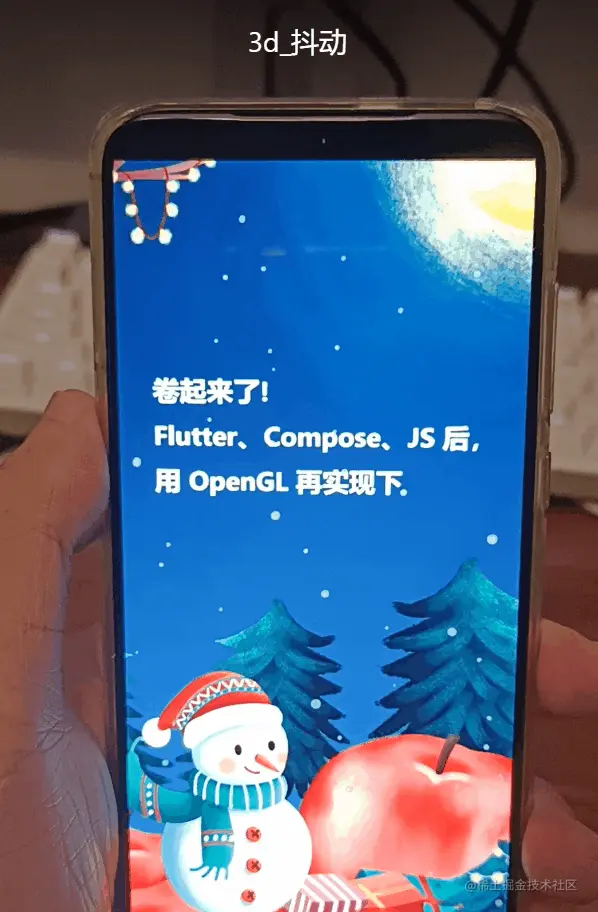``````private final SensorEventListener mSensorEventListener = new SensorEventListener() {
@Override
public void onSensorChanged(SensorEvent event) {
// 对传感器的数据追加低通滤波
if (event.sensor.getType() == Sensor.TYPE_ACCELEROMETER) {
mAcceleValues = lowPass(event.values.clone(), mAcceleValues);
}
if (event.sensor.getType() == Sensor.TYPE_MAGNETIC_FIELD) {
mMageneticValues = lowPass(event.values.clone(), mMageneticValues);
}

// ... 省略具体代码
// x轴的偏转角度
float degreeX = (float) Math.toDegrees(values);
// y轴的偏转角度
float degreeY = (float) Math.toDegrees(values);
// z轴的偏转角度
float degreeZ = (float) Math.toDegrees(values);

// 拿到 xy 轴的旋转角度，进行矩阵变换
updateMatrix(degreeX, degreeY);
}
};
``````## 参考 & 源码地址

1. 自如客APP裸眼3D效果的实现 @自如大前端团队

2. 拿去吧你！Flutter 仿自如 App 裸眼 3D 效果 @Nayuta

3. Compose版来啦！仿自如裸眼3D效果 @付十一

4. GitHub: Low-Pass-Filter-To-Android-Sensors

## 关于我

Hello，我是 却把清梅嗅 ，如果您觉得文章对您有价值，欢迎 ❤️，也欢迎关注我的 博客 或者 GitHub